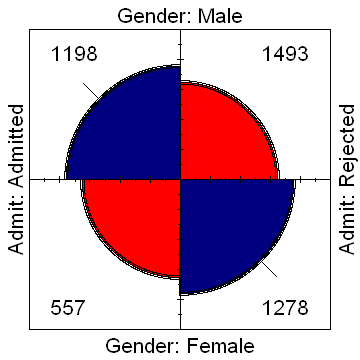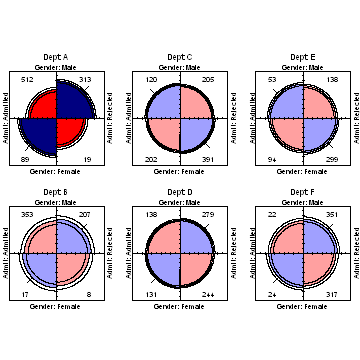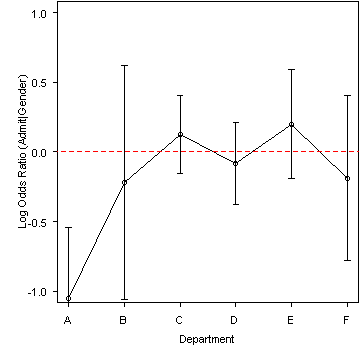# Output from berk-4fold.R

```library(vcd)
data("UCBAdmissions")

# rearrange dimensions
UCB <- aperm(UCBAdmissions, c(2, 1, 3))
# marginal table, collapsing over Dept
fourfold(margin.table(UCB, c(1, 2)))
``````# 3-way table, stratified by Dept
fourfold(UCB, mfrow = c(2, 3))
``````# test homogeneity of odds ratios over Dept
woolf_test(UCB)
```
```##
## 	Woolf-test on Homogeneity of Odds Ratios (no 3-Way assoc.)
##
## data:  UCB
## X-squared = 17.9, df = 5, p-value = 0.003072
```
```# calculate odds ratios
oddsratio(UCBAdmissions, log = FALSE)
```
```##      A      B      C      D      E      F
## 0.3492 0.8025 1.1331 0.9213 1.2216 0.8279
```
```# plot log odds ratios
plot(oddsratio(UCBAdmissions), xlab = "Department", ylab = "Log Odds Ratio (Admit|Gender)")
```Generated with `R version 2.15.1 (2012-06-22)` using the R package knitr (version `0.8.4`) on `Wed Sep 26 08:59:54 2012`.